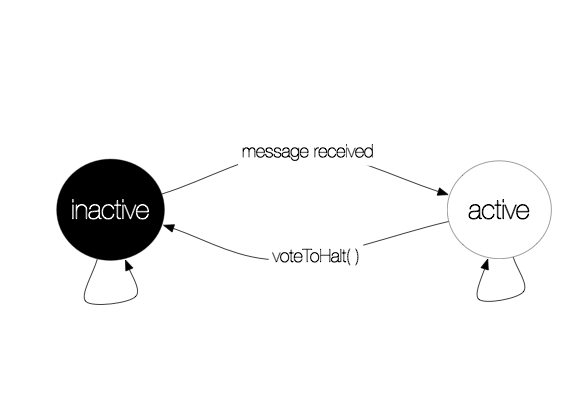#CodeTheWorld

Architecture

# Hadoop, part 3 : graph processing with Giraph

For the last part of this Hadoop series, I will introduce you the way to process graphs with Hadoop, through Apache Giraph.

We will first introduce Giraph, then we will take a look at how the graphs are processed, then we will implement a classical example : the Travelling Salesman Problem, in its brute force version.

### 1. Giraph

With the emergence of Facebook, LinkedIn, and the new social networks, the Big Data problems have lead us to Big Graph problems, as we try to process complex graphs, for example to obtain the shortest path between two nodes, or the friends of a friend, etc…
Hadoop and its eco-system is made to process large distributed sets of data, and Giraph follows this logic, and is a way to parallelize the treatment of a graph.

### 2. How it works

Let’s start by describing the way the graphs are represented through Giraph’s eyes.
The graphs are defined by all their vertexes, and four types are needed to define the graph :

-I is the id type
-V is the value type
-E is the type of the edge values
-M is the type of the messages

But what are those messages? The messages are at the root of Giraph’s way to process the Graph. Each vertex, when processed, can send a message to its connected vertexes.
The id is the unique identifier of the vertex, while the value is the value of the node, and the edge value is the “cost” of the edge between two vertexes.
You can create your own graphs, and ids can be lists, messages can be images, etc…Here is a basic vision of the vertexes from Giraph :Apart from these basic characteristics, Giraph adds another dimension to the graph processing : the vertexes can be active or inactive. They all begin the process as active, then they become inactive if they “vote to halt”
When they are inactive, they are not processed, and they can come back to activity only if they receive one (or more) message, and then they can be processed normally. The global treatment stops when all the vertexes are inactive. This scheme describes the transition from active to inactive, and the contrary.Giraph will the process the graph using “supersteps” : a superstep is a step during which the vertexes send messages to each other. Only the active vertexes are processed, and they call the method
`compute()` at each verticle, while they call the method `voteToHalt()` in order to be inactive. The process ends when all the vertexes vote to halt.

### 3. The Travelling Salesman Problem Example

To illustrate the way Giraph works, we implemented quickly the Travelling Salesman Problem (TSP), in its “brute-force” version. As a reminder, in the TSP, we try to find the shortest path between different cities that eaches each city once and comes back to the source city. The Graph modelisation in Giraph lets us complexify the original TSP :
-the edges are oriented, thus we the distance from city A to city B can be different to the distance from city B to city A
-the values of the vertexes are taken into account : it means that passing by a city also adds a distance
Now, we can then focus on how implement it on Giraph : we are then in possession of a graph representing cities, linked with asymetrical edges. At each superstep, Giraph will compute the active nodes : this node will send a new message to the remaining “cities”, in order to try all the possible paths, and during the last superstep, the source node will collect all the distances, and take the shortest.
Here is a little animation showing how it works :
The source code is available on gitHub here, and all the necessary instructions.

## Auteur/Autrice

•Ce site utilise Akismet pour réduire les indésirables. En savoir plus sur comment les données de vos commentaires sont utilisées.